# Fraction calculator

The calculator performs basic and advanced operations with fractions, expressions with fractions combined with integers, decimals, and mixed numbers. It also shows detailed step-by-step information about the fraction calculation procedure. Solve problems with two, three, or more fractions and numbers in one expression.

## Result:

### 1/3 * 1/3 = 1/9 ≅ 0.1111111

Spelled result in words is one ninth.

### How do you solve fractions step by step?

1. Multiple: 1/3 * 1/3 = 1 · 1/3 · 3 = 1/9
Multiply both numerators and denominators. Result fraction keep to lowest possible denominator GCD(1, 9) = 1. In the next intermediate step, the fraction result cannot be further simplified by canceling.
In words - one third multiplied by one third = one ninth.

#### Rules for expressions with fractions:

Fractions - use the slash “/” between the numerator and denominator, i.e., for five-hundredths, enter 5/100. If you are using mixed numbers, be sure to leave a single space between the whole and fraction part.
The slash separates the numerator (number above a fraction line) and denominator (number below).

Mixed numerals (mixed fractions or mixed numbers) write as non-zero integer separated by one space and fraction i.e., 1 2/3 (having the same sign). An example of a negative mixed fraction: -5 1/2.
Because slash is both signs for fraction line and division, we recommended use colon (:) as the operator of division fractions i.e., 1/2 : 3.

Decimals (decimal numbers) enter with a decimal point . and they are automatically converted to fractions - i.e. 1.45.

The colon : and slash / is the symbol of division. Can be used to divide mixed numbers 1 2/3 : 4 3/8 or can be used for write complex fractions i.e. 1/2 : 1/3.
An asterisk * or × is the symbol for multiplication.
Plus + is addition, minus sign - is subtraction and ()[] is mathematical parentheses.
The exponentiation/power symbol is ^ - for example: (7/8-4/5)^2 = (7/8-4/5)2

#### Examples:

subtracting fractions: 2/3 - 1/2
multiplying fractions: 7/8 * 3/9
dividing Fractions: 1/2 : 3/4
exponentiation of fraction: 3/5^3
fractional exponents: 16 ^ 1/2
adding fractions and mixed numbers: 8/5 + 6 2/7
dividing integer and fraction: 5 ÷ 1/2
complex fractions: 5/8 : 2 2/3
decimal to fraction: 0.625
Fraction to Decimal: 1/4
Fraction to Percent: 1/8 %
comparing fractions: 1/4 2/3
multiplying a fraction by a whole number: 6 * 3/4
square root of a fraction: sqrt(1/16)
reducing or simplifying the fraction (simplification) - dividing the numerator and denominator of a fraction by the same non-zero number - equivalent fraction: 4/22
expression with brackets: 1/3 * (1/2 - 3 3/8)
compound fraction: 3/4 of 5/7
fractions multiple: 2/3 of 3/5
divide to find the quotient: 3/5 ÷ 2/3

The calculator follows well-known rules for order of operations. The most common mnemonics for remembering this order of operations are:
PEMDAS - Parentheses, Exponents, Multiplication, Division, Addition, Subtraction.
BEDMAS - Brackets, Exponents, Division, Multiplication, Addition, Subtraction
BODMAS - Brackets, Of or Order, Division, Multiplication, Addition, Subtraction.
GEMDAS - Grouping Symbols - brackets (){}, Exponents, Multiplication, Division, Addition, Subtraction.
Be careful, always do multiplication and division before addition and subtraction. Some operators (+ and -) and (* and /) has the same priority and then must evaluate from left to right.

## Fractions in word problems:

• Write 3Write a real world problem involving the multiplication of a fraction and a whole number with a product that is between 8 and 10 then solve the problem
• Multiplying fractionsWhat is 1/3 of 5?
• Visit to grandfatherShane's family traveled 3/10 of the distance to his grandfather’s house on Saturday. They traveled 4/7 of the remaining distance on Sunday. What fraction of the total distance to his grandfather’s house was traveled on Sunday?
• Sign of a expressionWhat is the sign of expression: minus 18 start fraction 9 divided by 17 end fraction plus left parenthesis minus 18 start fraction 9 divided by 17 end fraction right parenthesis?
• Lengths of the poolMiguel swam 6 lengths of the pool. Mat swam 3 times as far as Miguel. Lionel swam 1/3 as far as Miguel. How many lengths did Mat swim?To three-eighths of one third, we add five quarters of one half and multiply the sum by four. How much will we get?
• A newspaper'sA newspaper's cover page is 3/8 text, and photographs fill the rest. If 2/5 of the text is an article about endangered species, what fraction of the whole cover page is the article about endangered species?
• Icecreams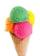Karthik bought 30 icecreams for his birthday .2/5 of them are chocolate icecreams. Find the number of chocolate icecreams he bought?
• The kennel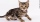20 dogs. Ratio dogs to cats is 3:2  how many cats?
• The number 4Two-thirds of a number is negative six. Find the number.
• Sally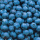Sally is making 15 individual blueberry cheesecakes. How many pounds of blueberries will be in each cheesecake if 5 1/4 pounds of blueberries are divided equally among them?
• Summerjobs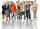The agency give summerjobs for 2352 students in 2018. The eighth was high-school students, the rest was undergraduates. How many undergraduates works via agency in 2018?
• A large 2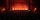A large popcorn bag holds four times as much as a small popcorn bag at the end of the party 3 1/3 small bags and 2 1/4 large bags left. How many small bags with the leftover popcorn fill?